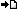RegisterMember LoginForgot Password ?? PHP , ASP , ASP.NET, VB.NET, C#, Java , jQuery , Android , iOS , Windows Phone

## Registered : 109,007

 HOME > VBScript Manual > Looping Through Code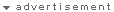Microsoft® Visual Basic® Scripting Edition Looping Through Code VBScript TutorialPreviousNext

##### Using Loops to Repeat Code
Looping allows you to run a group of statements repeatedly. Some loops repeat statements until a condition is False; others repeat statements until a condition is True. There are also loops that repeat statements a specific number of times.

The following looping statements are available in VBScript:

• Do...Loop: Loops while or until a condition is True.
• While...Wend: Loops while a condition is True.
• For...Next: Uses a counter to run statements a specified number of times.
• For Each...Next: Repeats a group of statements for each item in a collection or each element of an array.
##### Using Do Loops
You can use Do...Loop statements to run a block of statements an indefinite number of times. The statements are repeated either while a condition is True or until a condition becomes True.
##### Repeating Statements While a Condition is True
Use the While keyword to check a condition in a Do...Loop statement. You can check the condition before you enter the loop (as shown in the following ChkFirstWhile example), or you can check it after the loop has run at least once (as shown in the ChkLastWhile example). In the ChkFirstWhile procedure, if myNum is set to 9 instead of 20, the statements inside the loop will never run. In the ChkLastWhile procedure, the statements inside the loop run only once because the condition is already False.
``` Sub ChkFirstWhile()
Dim counter, myNum
counter = 0
myNum = 20
Do While myNum > 10
myNum = myNum - 1
counter = counter + 1
Loop
MsgBox "The loop made " & counter & " repetitions."
End Sub

Sub ChkLastWhile()
Dim counter, myNum
counter = 0
myNum = 9
Do
myNum = myNum - 1
counter = counter + 1
Loop While myNum > 10
MsgBox "The loop made " & counter & " repetitions."
End Sub
```
##### Repeating a Statement Until a Condition Becomes True
You can use the Until keyword in two ways to check a condition in a Do...Loop statement. You can check the condition before you enter the loop (as shown in the following ChkFirstUntil example), or you can check it after the loop has run at least once (as shown in the ChkLastUntil example). As long as the condition is False, the looping occurs.
``` Sub ChkFirstUntil()
Dim counter, myNum
counter = 0
myNum = 20
Do Until myNum = 10
myNum = myNum - 1
counter = counter + 1
Loop
MsgBox "The loop made " & counter & " repetitions."
End Sub

Sub ChkLastUntil()
Dim counter, myNum
counter = 0
myNum = 1
Do
myNum = myNum + 1
counter = counter + 1
Loop Until myNum = 10
MsgBox "The loop made " & counter & " repetitions."
End Sub
```
##### Exiting a Do...Loop Statement from Inside the Loop
You can exit a Do...Loop by using the Exit Do statement. Because you usually want to exit only in certain situations, such as to avoid an endless loop, you should use the Exit Do statement in the True statement block of an If...Then...Else statement. If the condition is False, the loop runs as usual.

In the following example, myNum is assigned a value that creates an endless loop. The If...Then...Else statement checks for this condition, preventing the endless repetition.

``` Sub ExitExample()
Dim counter, myNum
counter = 0
myNum = 9
Do Until myNum = 10
myNum = myNum - 1
counter = counter + 1
If myNum < 10 Then Exit Do
Loop
MsgBox "The loop made " & counter & " repetitions."
End Sub
```
##### Using While...Wend
The While...Wend statement is provided in VBScript for those who are familiar with its usage. However, because of the lack of flexibility in While...Wend, it is recommended that you use Do...Loop instead.
##### Using For...Next
You can use For...Next statements to run a block of statements a specific number of times. For loops, use a counter variable whose value is increased or decreased with each repetition of the loop.

For example, the following procedure causes a procedure called MyProc to execute 50 times. The For statement specifies the counter variable x and its start and end values. The Next statement increments the counter variable by 1.

``` Sub DoMyProc50Times()
Dim x
For x = 1 To 50
MyProc
Next
End Sub
```
Using the Step keyword, you can increase or decrease the counter variable by the value you specify. In the following example, the counter variable j is incremented by 2 each time the loop repeats. When the loop is finished, total is the sum of 2, 4, 6, 8, and 10.
``` Sub TwosTotal()
Dim j, total
For j = 2 To 10 Step 2
total = total + j
Next
MsgBox "The total is " & total
End Sub
```
To decrease the counter variable, you use a negative Step value. You must specify an end value that is less than the start value. In the following example, the counter variable myNum is decreased by 2 each time the loop repeats. When the loop is finished, total is the sum of 16, 14, 12, 10, 8, 6, 4, and 2.

``` Sub NewTotal()
Dim myNum, total
For myNum = 16 To 2 Step -2
total = total + myNum
Next
MsgBox "The total is " & total
End Sub
```
You can exit any For...Next statement before the counter reaches its end value by using the Exit For statement. Because you usually want to exit only in certain situations, such as when an error occurs, you should use the Exit For statement in the True statement block of an If...Then...Else statement. If the condition is False, the loop runs as usual.

##### Using For Each...Next
A For Each...Next loop is similar to a For...Next loop. Instead of repeating the statements a specified number of times, a For Each...Next loop repeats a group of statements for each item in a collection of objects or for each element of an array. This is especially helpful if you don't know how many elements are in a collection.

In the following HTML code example, the contents of a Dictionary object is used to place text in several text boxes:

``` <HTML>
<SCRIPT LANGUAGE="VBScript">
<!--
Sub cmdChange_OnClick
Dim d                   'Create a variable
Set d = CreateObject("Scripting.Dictionary")

For Each I in d
Document.frmForm.Elements(I).Value = D.Item(I)
Next
End Sub
-->
</SCRIPT>
<BODY>
<CENTER>
<FORM NAME="frmForm"

<Input Type = "Text"><p>
<Input Type = "Text"><p>
<Input Type = "Text"><p>
<Input Type = "Text"><p>
</FORM>
</CENTER>
</BODY>
</HTML>
```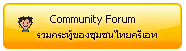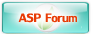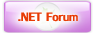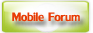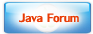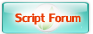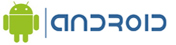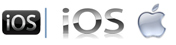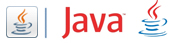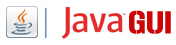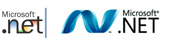Free Tutorial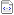สอน Google Maps Apiสอน Windows Serviceสอน Entity Frameworkสอน Androidสอน Java เขียน JavaJava GUI Swingสอน JSP (Web App)iOS (iPhone,iPad)Windows PhoneWindows AzureWindows StoreLaravel FrameworkYii PHP Frameworkสอน jQueryสอน jQuery กับ Ajaxสอน PHP OOP (Vdo)Ajax Tutorials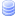SQL Tutorialsสอน SQL (Part 2)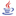JavaScript TutorialJavascript TipsVBScript TutorialVBScript ValidationMicrosoft Access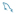MySQL Tutorials-- Stored ProcedureMariaDB Database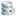SQL Server TutorialSQL Server 2005SQL Server 2008SQL Server 2012-- Stored ProcedureOracle Database-- Stored ProcedureSVN (Subversion)แนวทางการทำ SEOปรับแต่งเว็บให้โหลดเร็วสุดยอด Source Code V2.0แจ้งชำระเงิน/โอนเงินลองค้นหาสิ่งที่ต้องการLoad balance : Server 03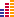© www.ThaiCreate.Com. 2003-2023 All Rights Reserved. ไทยครีเอทบริการ จัดทำดูแลแก้ไข Web Application ทุกรูปแบบ (PHP, .Net Application, VB.Net, C#) [Conditions Privacy Statement] ติดต่อโฆษณา 081-987-6107 อัตราราคา คลิกที่นี่